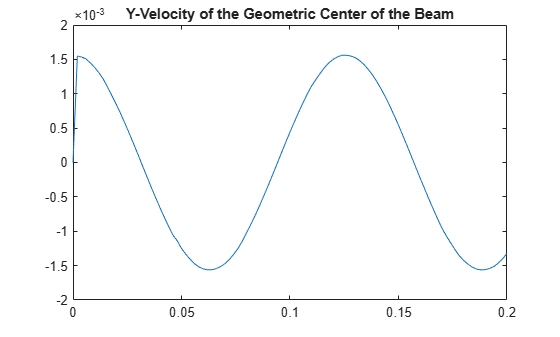# interpolateVelocity

Interpolate velocity at arbitrary spatial locations for all time or frequency steps for dynamic structural model

## Syntax

``intrpVel = interpolateVelocity(structuralresults,xq,yq)``
``intrpVel = interpolateVelocity(structuralresults,xq,yq,zq)``
``intrpVel = interpolateVelocity(structuralresults,querypoints)``

## Description

````intrpVel = interpolateVelocity(structuralresults,xq,yq)` returns the interpolated velocity values at the 2-D points specified in `xq` and `yq` for all time or frequency steps.```

example

````intrpVel = interpolateVelocity(structuralresults,xq,yq,zq)` uses the 3-D points specified in `xq`, `yq`, and `zq`.```
````intrpVel = interpolateVelocity(structuralresults,querypoints)` uses the points specified in `querypoints`.```

## Examples

collapse all

Interpolate velocity at the geometric center of a beam under a harmonic excitation.

Create a transient dynamic model for a 3-D problem.

`structuralmodel = createpde("structural","transient-solid");`

Create the geometry and include it in the model. Plot the geometry.

```gm = multicuboid(0.06,0.005,0.01); structuralmodel.Geometry = gm; pdegplot(structuralmodel,"FaceLabels","on","FaceAlpha",0.5) view(50,20)```Specify Young's modulus, Poisson's ratio, and the mass density of the material.

```structuralProperties(structuralmodel,"YoungsModulus",210E9, ... "PoissonsRatio",0.3, ... "MassDensity",7800);```

Fix one end of the beam.

`structuralBC(structuralmodel,"Face",5,"Constraint","fixed");`

Apply a sinusoidal displacement along the `y`-direction on the end opposite the fixed end of the beam.

```structuralBC(structuralmodel,"Face",3, ... "YDisplacement",1E-4, ... "Frequency",50);```

Generate a mesh.

`generateMesh(structuralmodel,"Hmax",0.01);`

Specify the zero initial displacement and velocity.

```structuralIC(structuralmodel,"Displacement",[0;0;0], ... "Velocity",[0;0;0]);```

Solve the model.

```tlist = 0:0.002:0.2; structuralresults = solve(structuralmodel,tlist);```

Interpolate velocity at the geometric center of the beam.

```coordsMidSpan = [0;0;0.005]; intrpVel = interpolateVelocity(structuralresults,coordsMidSpan);```

Plot the `y`-component of velocity of the geometric center of the beam.

```figure plot(structuralresults.SolutionTimes,intrpVel.vy) title("Y-Velocity of the Geometric Center of the Beam")```## Input Arguments

collapse all

Solution of the dynamic structural analysis problem, specified as a `TransientStructuralResults` or `FrequencyStructuralResults` object. Create `structuralresults` by using the `solve` function.

Example: ```structuralresults = solve(structuralmodel,tlist)```

x-coordinate query points, specified as a real array. `interpolateVelocity` evaluates velocities at the 2-D coordinate points `[xq(i),yq(i)]` or at the 3-D coordinate points `[xq(i),yq(i),zq(i)]`. Therefore, `xq`, `yq`, and (if present) `zq` must have the same number of entries.

`interpolateVelocity` converts query points to column vectors `xq(:)`, `yq(:)`, and (if present) `zq(:)`. It returns velocities as an `FEStruct` object with the properties containing vectors of the same size as these column vectors. To ensure that the dimensions of the returned solution are consistent with the dimensions of the original query points, use the `reshape` function. For example, use ```intrpVel = reshape(intrpVel.ux,size(xq))```.

Data Types: `double`

y-coordinate query points, specified as a real array. `interpolateVelocity` evaluates velocities at the 2-D coordinate points `[xq(i),yq(i)]` or at the 3-D coordinate points `[xq(i),yq(i),zq(i)]`. Therefore, `xq`, `yq`, and (if present) `zq` must have the same number of entries. Internally, `interpolateVelocity` converts query points to the column vector `yq(:)`.

Data Types: `double`

z-coordinate query points, specified as a real array. `interpolateVelocity` evaluates velocities at the 3-D coordinate points `[xq(i),yq(i),zq(i)]`. Therefore, `xq`, `yq`, and `zq` must have the same number of entries. Internally, `interpolateVelocity` converts query points to the column vector `zq(:)`.

Data Types: `double`

Query points, specified as a real matrix with either two rows for 2-D geometry or three rows for 3-D geometry. `interpolateVelocity` evaluates velocities at the coordinate points `querypoints(:,i)`, so each column of `querypoints` contains exactly one 2-D or 3-D query point.

Example: For 2-D geometry, ```querypoints = [0.5,0.5,0.75,0.75; 1,2,0,0.5]```

Data Types: `double`

## Output Arguments

collapse all

Velocities at the query points, returned as an `FEStruct` object with the properties representing spatial components of velocity at the query points. For query points that are outside the geometry, `intrpVel` returns `NaN`. Properties of an `FEStruct` object are read-only.

## Version History

Introduced in R2018a